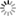Personal tools
You are here: Home Statistics

Statistics

- Modern Statistics

Statistics is both a body of theory and methods of analysis. The subject matters of statistics covers a wide range - extending from the planning of experiments and other studies that generate data to the collection, analysis, presentation, and interpretation of the data. Numerical data constitute the raw material of the subject matter of statistics.

The essence of modern statistics, however, is the theory and the methodology of drawing inferences that extend beyond the particular set of data examined and of making decisions based on appropriate analysis of such inferential data

- Probability versus Statistics

Probability and statistics are related areas of mathematics which concern themselves with analyzing the relative frequency of events. Still, there are fundamental differences in the way they see the world:

• Probability deals with predicting the likelihood of future events, while statistics involves the analysis of the frequency of past events.
• Probability is primarily a theoretical branch of mathematics, which studies the consequences of mathematical definitions. Statistics is primarily an applied branch of mathematics, which tries to make sense of observations in the real world.

Both subjects are important, relevant, and useful. But they are different, and understanding the distinction is crucial in properly interpreting the relevance of mathematical evidence. Many a gambler has gone to a cold and lonely grave for failing to make the proper distinction between probability and statistics.

This distinction will perhaps become clearer if we trace the thought process of a mathematician encountering her first craps game:

• If this mathematician were a probabilist, she would see the dice and think ``Six-sided dice? Presumably each face of the dice is equally likely to land face up. Now assuming that each face comes up with probability 1/6, I can figure out what my chances of crapping out are.''
• If instead a statistician wandered by, she would see the dice and think ``Those dice may look OK, but how do I know that they are not loaded? I'll watch a while, and keep track of how often each number comes up. Then I can decide if my observations are consistent with the assumption of equal-probability faces. Once I'm confident enough that the dice are fair, I'll call a probabilist to tell me how to play.''

In summary, probability theory enables us to find the consequences of a given ideal world, while statistical theory enables us to to measure the extent to which our world is ideal.

- Statistics and Machine Learning

Statistics and machine learning are two very closely related fields.

In fact, the line between the two can be very fuzzy at times. Nevertheless, there are methods that clearly belong to the field of statistics that are not only useful, but invaluable when working on a machine learning project.

It would be fair to say that statistical methods are required to effectively work through a machine learning predictive modeling project.

[More to come ...]

Document Actions